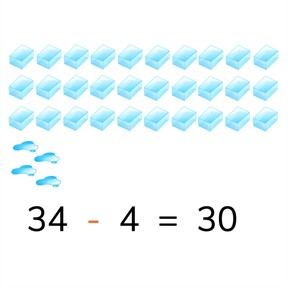Subtracting numbers to 100 to a tens number

# Subtracting numbers to 100 to a tens number

No account needed.8,000 schools use Gynzy92,000 teachers use Gynzy1,600,000 students use Gynzy

## General

Students learn to subtract numbers to 100 to a tens number

2.NBT.B.5

## Relevance

Discuss with students that it is important to be able to subtract numbers to 100, so you can determine how much you have left.

## Introduction

Show a subtraction problem on the interactive whiteboard. A portion has been completed, ask the students which number is missing. Use the image as support- students can count that. Repeat this exercise with the blocks. Then ask students to determine which of the students on the interactive whiteboard has the correct answer.

## Development

Start by reminding students that tens are a group of ten and have a zero in the ones place. The lesson is presented in visual form, in the abstract, and in story form. You can select which method best suits your class by clicking on the blue menu in the bottom right. The lesson starts with a visual explanation. Tell students that they can subtract by counting. First count how many there are in total, this is the first number of the subtraction problem (the minuend). Then count how many are taken away, this is the second number of the subtraction problem (the subtrahend). When you take the first away from the second you find the difference. Show this on the interactive whiteboard using the banana and cherries. Check that students are able to do this by asking them to take away the blocks shown on the interactive whiteboard. Next you explain a different type of subtraction problem. Tell students that you first look at how many objects there are in total, that is the first number of the subtraction problem (the minuend). Then you look at what the difference is. You then determine how many need to be taken away. Explain to students that you can subtract different kinds of problems in different ways. You can use a number line, for example. You can also choose to make a different subtraction problem in which you take the difference away from the total number of objects (or minuend) to find what the second number (or subtrahend) should be. Ask students to solve a few problems and ask them to name their strategy. Be sure to emphasize that there is no incorrect strategy, but that students should use the strategy they feel works best in a given situation. Finally walk through the steps of solving a story problem with the students. Remind them that they must first determine what kind of math problem it is, determine which numbers are important for the story problem, say or write the problem and then solve. Do a story problem together as a class and then ask the students to solve a few story problems individually or in pairs.

To check that students are able to solve subtraction problem with numbers to 100 to a tens number you can ask the following questions:
- What are the steps of solving a story problem?
- How do you calculate 57 - 7 and 84 - ..... = 40

## Guided practice

Students are given a variety of subtraction problems including problems with visual support, problems in the abstract and subtraction story problems.

## Closing

Discuss with students that it is important to be able to subtract numbers to 100 to a tens number, so you can easily determine how much you have left. Show the money on the interactive whiteboard and ask which number is missing in the subtraction problem. Have students explain their strategy. Then students must solve a subtraction problem on the number line.

## Teaching tips

Students who have difficulty subtracting numbers to 100 to a tens number can use the number line as visual support. Remind them that they can take different jumps on the number line.

### The online teaching platform for interactive whiteboards and displays in schools

• Save time building lessons

• Manage the classroom more efficiently

• Increase student engagement